### Econ100B Winter2010 Midterm 1 Long Probmem 1 Gorry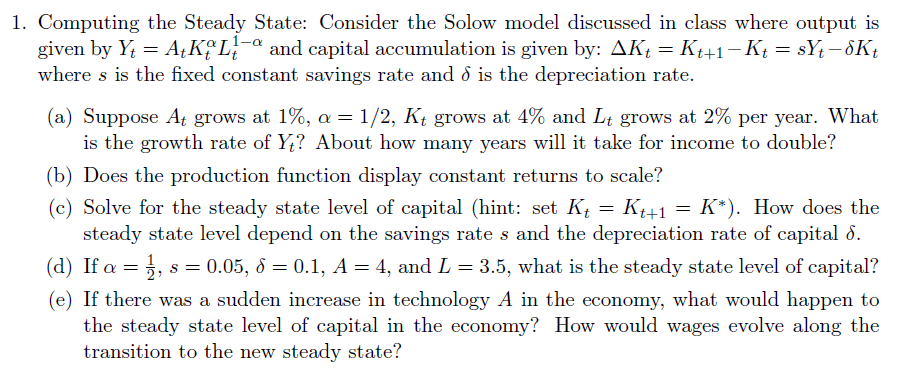(a) With production function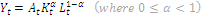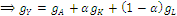Plugging in the growth rates given to us,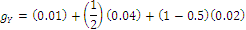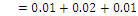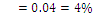Production grows at 4%. How many years will it take for production to double? Use the “rule of 70”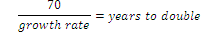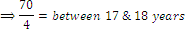It’ll take between 17 and 18 years for production to double with a growth rate of 4% *Remember that you won’t have the use of a calculator on the exam… If we assume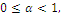then our production function is a Cobb-Douglas function with constant returns to scale. Constant returns to production come from the exponents above K and L summing to one. (c) - page 102, particularly page 105 The incantation of a steady state is the following “A steady state is a value,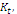such that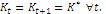This implies that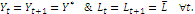(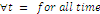) Take the two equations above, and establish the steady state. Equation One – the Capital Accumulation Equation  (aka, the Law of Motion of Capital)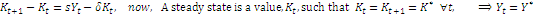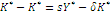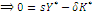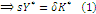Equation Two – The production function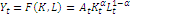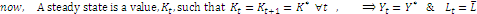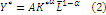Now – combine (1)  & (2), and solve for Capital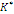,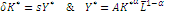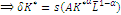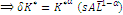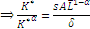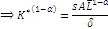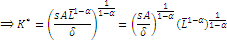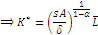You can see that the steady state level of capital (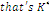) depends on all the stuff in the right-hand-side;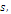the savings rate. If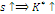, the depreciation rate. If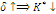(d) – plugging the parameter values given into our steady state level of capital equation,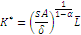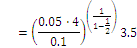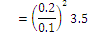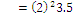You can do this without a calculator – just be very careful!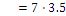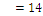The steady state level of capital is fourteen. Where K-star is the steady state level of capital. Starting off at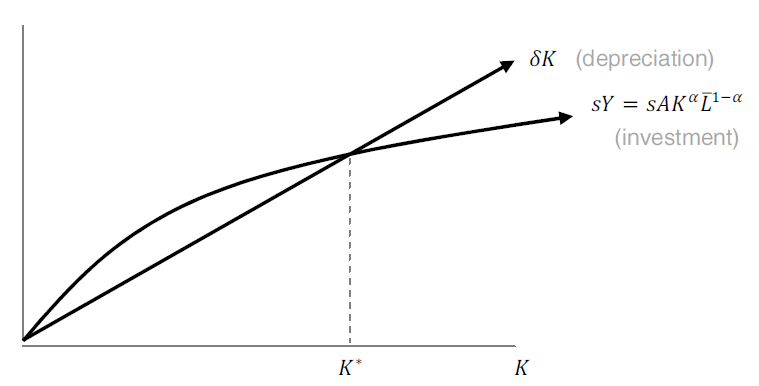A sudden decrease in A would lower investment curve, decreasing the steady state of capital.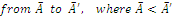The steady state level of capital will decrease.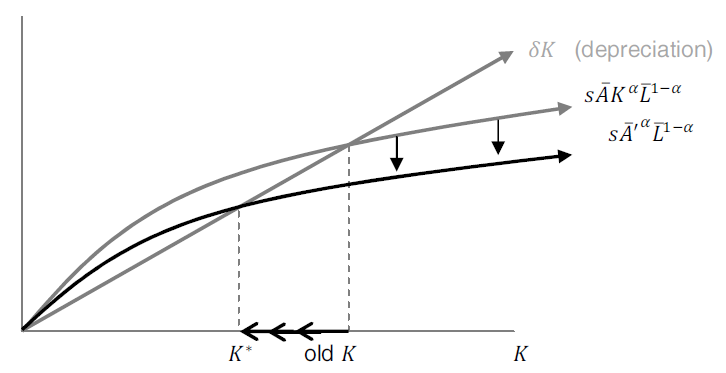Wages?  I’m going to interpret the question, “how do wages evolve…?” as, “will wages increase or decrease?” Recall that wages come from the resource constraint equation,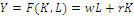First Order Conditions,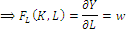Okay, so wages will work out to be how our production function changes as the quantity of labor changes (or, more simply, the first derivative of our production function with respect to labor).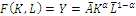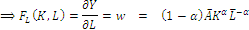Great, wages are equal to the equation above. With (1-alpha) being positive, if we were to decrease A-bar, that will decrease wages.
Comments